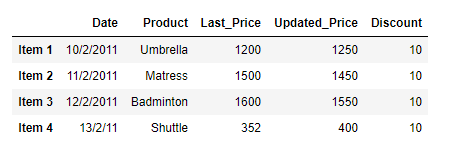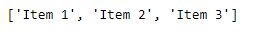# Return the Index label if some condition is satisfied over a column in Pandas Dataframe

Given a Dataframe, return all those index labels for which some condition is satisfied over a specific column. Solution #1: We can use simple indexing operation to select all those values in the column which satisfies the given condition.

## Python3

 `# importing pandas as pd` `import` `pandas as pd`   `# Create the dataframe` `df ``=` `pd.DataFrame({``'Date'``:[``'10/2/2011'``, ``'11/2/2011'``, ``'12/2/2011'``, ``'13/2/2011'``],` `                   ``'Product'``:[``'Umbrella'``, ``'Mattress'``, ``'Badminton'``, ``'Shuttle'``],` `                   ``'Last_Price'``:[``1200``, ``1500``, ``1600``, ``352``],` `                   ``'Updated_Price'``:[``1250``, ``1450``, ``1550``, ``400``],` `                   ``'Discount'``:[``10``, ``10``, ``10``, ``10``]})`   `# Create the indexes` `df.index ``=``[``'Item 1'``, ``'Item 2'``, ``'Item 3'``, ``'Item 4'``]`   `# Print the dataframe` `print``(df)`

Output :Now, we want to find out the index labels of all items whose ‘Updated_Price’ is greater than 1000.

## Python3

 `# Select all the rows which satisfies the criteria` `# convert the collection of index labels to list.` `Index_label ``=` `df[df[``'Updated Price'``]>``1000``].index.tolist()`   `# Print all the labels` `print``(Index_label)`

Output :As we can see in the output, the above operation has successfully evaluated all the values and has returned a list containing the index labels.   Solution #2: We can use Pandas Dataframe.query() function to select all the rows which satisfies some condition over a given column.

## Python3

 `# importing pandas as pd` `import` `pandas as pd`   `# Create the dataframe` `df ``=` `pd.DataFrame({``'Date'``:[``'10/2/2011'``, ``'11/2/2011'``, ``'12/2/2011'``, ``'13/2/2011'``],` `                   ``'Product'``:[``'Umbrella'``, ``'Mattress'``, ``'Badminton'``, ``'Shuttle'``],` `                   ``'Last_Price'``:[``1200``, ``1500``, ``1600``, ``352``],` `                   ``'Updated_Price'``:[``1250``, ``1450``, ``1550``, ``400``],` `                   ``'Discount'``:[``10``, ``10``, ``10``, ``10``]})`   `# Create the indexes` `df.index ``=``[``'Item 1'``, ``'Item 2'``, ``'Item 3'``, ``'Item 4'``]`   `# Print the dataframe` `print``(df)`

Output :Now, we want to find out the index labels of all items whose ‘Updated_Price’ is greater than 1000.

## Python3

 `# Select all the rows which satisfies the criteria` `# convert the collection of index labels to list.` `Index_label ``=` `df.query(``'Updated_Price > 1000'``).index.tolist()`   `# Print all the labels` `print``(Index_label)`

Output :Whether you're preparing for your first job interview or aiming to upskill in this ever-evolving tech landscape, GeeksforGeeks Courses are your key to success. We provide top-quality content at affordable prices, all geared towards accelerating your growth in a time-bound manner. Join the millions we've already empowered, and we're here to do the same for you. Don't miss out - check it out now!

Previous
Next